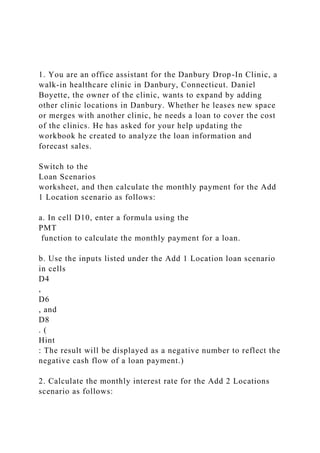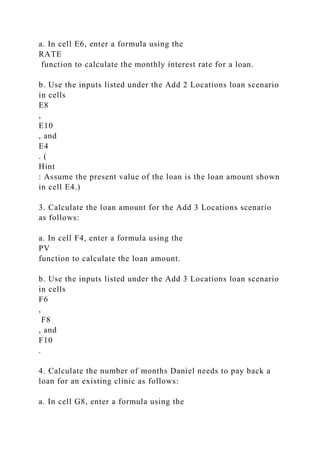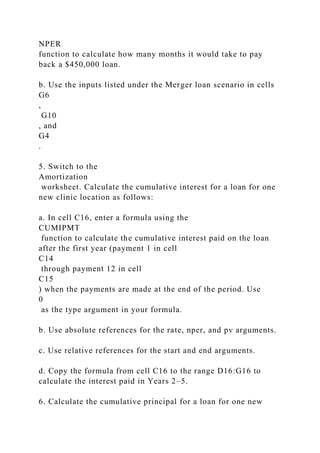Diese Präsentation wurde erfolgreich gemeldet.

# 1. You are an office assistant for the Danbury Drop-In Clinic, a.docx

Anzeige
Anzeige
Anzeige
Anzeige
Anzeige
Anzeige
Anzeige
Anzeige
Anzeige
Anzeige
AnzeigeAnzeige
×

1 von 9 Anzeige

# 1. You are an office assistant for the Danbury Drop-In Clinic, a.docx

1. You are an office assistant for the Danbury Drop-In Clinic, a walk-in healthcare clinic in Danbury, Connecticut. Daniel Boyette, the owner of the clinic, wants to expand by adding other clinic locations in Danbury. Whether he leases new space or merges with another clinic, he needs a loan to cover the cost of the clinics. He has asked for your help updating the workbook he created to analyze the loan information and forecast sales.

Switch to the
Loan Scenarios
worksheet, and then calculate the monthly payment for the Add 1 Location scenario as follows:

a. In cell D10, enter a formula using the
PMT
function to calculate the monthly payment for a loan.

b. Use the inputs listed under the Add 1 Location loan scenario in cells
D4
,
D6
, and
D8
. (
Hint
: The result will be displayed as a negative number to reflect the negative cash flow of a loan payment.)

2. Calculate the monthly interest rate for the Add 2 Locations scenario as follows:

a. In cell E6, enter a formula using the
RATE
function to calculate the monthly interest rate for a loan.

b. Use the inputs listed under the Add 2 Locations loan scenario in cells
E8
,
E10
, and
E4
. (
Hint
: Assume the present value of the loan is the loan amount shown in cell E4.)

3. Calculate the loan amount for the Add 3 Locations scenario as follows:

a. In cell F4, enter a formula using the
PV
function to calculate the loan amount.

b. Use the inputs listed under the Add 3 Locations loan scenario in cells
F6
,
F8
, and
F10
.

4. Calculate the number of months Daniel needs to pay back a loan for an existing clinic as follows:

a. In cell G8, enter a formula using the
NPER
function to calculate how many months it would take to pay back a \$450,000 loan.

b. Use the inputs listed under the Merger loan scenario in cells
G6
,
G10
, and
G4
.

5. Switch to the
Amortization
worksheet. Calculate the cumulative interest for a loan for one new clinic location as follows:

a. In cell C16, enter a formula using the
CUMIPMT
function to calculate the cumulative interest paid on the loan after the first year (payment 1 in cell
C14
through payment 12 in cell
C15
) when the payments are made at the end of the period. Use
0
as the type argument in your formula.

b. Use absolute references for the rate, nper, and pv arguments.

c. Use relative references for the start and end arguments.

d. Copy the formula from cell C16 to the range D16:G16 to calculate the interest paid in Years 2–5.

6. Calculate the cumulative principal for a loan for one new clinic location as follows:

a. In cell C17, enter a formula using the
CUMPRINC
function to calculate the cumulative principal paid in the first year (payment 1 in cell
C14
through payment 12 in cell
C15
) when the payments are made at the end of the period. Use
0
as the type argument in your formula.

b. Use absolute references for the rate, nper, and pv arguments.

c. Use relative references for the start and end arguments.

d. Copy.

1. You are an office assistant for the Danbury Drop-In Clinic, a walk-in healthcare clinic in Danbury, Connecticut. Daniel Boyette, the owner of the clinic, wants to expand by adding other clinic locations in Danbury. Whether he leases new space or merges with another clinic, he needs a loan to cover the cost of the clinics. He has asked for your help updating the workbook he created to analyze the loan information and forecast sales.

Switch to the
Loan Scenarios
worksheet, and then calculate the monthly payment for the Add 1 Location scenario as follows:

a. In cell D10, enter a formula using the
PMT
function to calculate the monthly payment for a loan.

b. Use the inputs listed under the Add 1 Location loan scenario in cells
D4
,
D6
, and
D8
. (
Hint
: The result will be displayed as a negative number to reflect the negative cash flow of a loan payment.)

2. Calculate the monthly interest rate for the Add 2 Locations scenario as follows:

a. In cell E6, enter a formula using the
RATE
function to calculate the monthly interest rate for a loan.

b. Use the inputs listed under the Add 2 Locations loan scenario in cells
E8
,
E10
, and
E4
. (
Hint
: Assume the present value of the loan is the loan amount shown in cell E4.)

3. Calculate the loan amount for the Add 3 Locations scenario as follows:

a. In cell F4, enter a formula using the
PV
function to calculate the loan amount.

b. Use the inputs listed under the Add 3 Locations loan scenario in cells
F6
,
F8
, and
F10
.

4. Calculate the number of months Daniel needs to pay back a loan for an existing clinic as follows:

a. In cell G8, enter a formula using the
NPER
function to calculate how many months it would take to pay back a \$450,000 loan.

b. Use the inputs listed under the Merger loan scenario in cells
G6
,
G10
, and
G4
.

5. Switch to the
Amortization
worksheet. Calculate the cumulative interest for a loan for one new clinic location as follows:

a. In cell C16, enter a formula using the
CUMIPMT
function to calculate the cumulative interest paid on the loan after the first year (payment 1 in cell
C14
through payment 12 in cell
C15
) when the payments are made at the end of the period. Use
0
as the type argument in your formula.

b. Use absolute references for the rate, nper, and pv arguments.

c. Use relative references for the start and end arguments.

d. Copy the formula from cell C16 to the range D16:G16 to calculate the interest paid in Years 2–5.

6. Calculate the cumulative principal for a loan for one new clinic location as follows:

a. In cell C17, enter a formula using the
CUMPRINC
function to calculate the cumulative principal paid in the first year (payment 1 in cell
C14
through payment 12 in cell
C15
) when the payments are made at the end of the period. Use
0
as the type argument in your formula.

b. Use absolute references for the rate, nper, and pv arguments.

c. Use relative references for the start and end arguments.

d. Copy.

Anzeige
Anzeige

### 1. You are an office assistant for the Danbury Drop-In Clinic, a.docx

1. 1. 1. You are an office assistant for the Danbury Drop-In Clinic, a walk-in healthcare clinic in Danbury, Connecticut. Daniel Boyette, the owner of the clinic, wants to expand by adding other clinic locations in Danbury. Whether he leases new space or merges with another clinic, he needs a loan to cover the cost of the clinics. He has asked for your help updating the workbook he created to analyze the loan information and forecast sales. Switch to the Loan Scenarios worksheet, and then calculate the monthly payment for the Add 1 Location scenario as follows: a. In cell D10, enter a formula using the PMT function to calculate the monthly payment for a loan. b. Use the inputs listed under the Add 1 Location loan scenario in cells D4 , D6 , and D8 . ( Hint : The result will be displayed as a negative number to reflect the negative cash flow of a loan payment.) 2. Calculate the monthly interest rate for the Add 2 Locations scenario as follows:
2. 2. a. In cell E6, enter a formula using the RATE function to calculate the monthly interest rate for a loan. b. Use the inputs listed under the Add 2 Locations loan scenario in cells E8 , E10 , and E4 . ( Hint : Assume the present value of the loan is the loan amount shown in cell E4.) 3. Calculate the loan amount for the Add 3 Locations scenario as follows: a. In cell F4, enter a formula using the PV function to calculate the loan amount. b. Use the inputs listed under the Add 3 Locations loan scenario in cells F6 , F8 , and F10 . 4. Calculate the number of months Daniel needs to pay back a loan for an existing clinic as follows: a. In cell G8, enter a formula using the
3. 3. NPER function to calculate how many months it would take to pay back a \$450,000 loan. b. Use the inputs listed under the Merger loan scenario in cells G6 , G10 , and G4 . 5. Switch to the Amortization worksheet. Calculate the cumulative interest for a loan for one new clinic location as follows: a. In cell C16, enter a formula using the CUMIPMT function to calculate the cumulative interest paid on the loan after the first year (payment 1 in cell C14 through payment 12 in cell C15 ) when the payments are made at the end of the period. Use 0 as the type argument in your formula. b. Use absolute references for the rate, nper, and pv arguments. c. Use relative references for the start and end arguments. d. Copy the formula from cell C16 to the range D16:G16 to calculate the interest paid in Years 2–5. 6. Calculate the cumulative principal for a loan for one new
4. 4. clinic location as follows: a. In cell C17, enter a formula using the CUMPRINC function to calculate the cumulative principal paid in the first year (payment 1 in cell C14 through payment 12 in cell C15 ) when the payments are made at the end of the period. Use 0 as the type argument in your formula. b. Use absolute references for the rate, nper, and pv arguments. c. Use relative references for the start and end arguments. d. Copy the formula from cell C17 to the range D17:G17 to calculate the principal paid in Years 2–5. 7. In cell H17, use the Error Checking command to identify the error in the cell, and then correct the error. ( Hint : The formula in the cell should calculate the total values in C17:G17 using the SUM function.) 8. Calculate the principal amounts in the loan amortization schedule as follows: a. In cell E22, enter a formula using the PPMT function to determine the amount of the first loan payment devoted to principal.
5. 5. b. Use absolute references only for the rate, nper, and pv arguments. c. Use cell A22 as the current period. (Hint : The period is based on a monthly payment schedule.) d. Copy the formula from cell E22 to the range E23:E81 to calculate the principal paid in Periods 2–60. 9. Calculate the interest amounts in the loan amortization schedule as follows: a. In cell F22, enter a formula using the IPMT function to determine the amount of the first loan payment devoted to interest. b. Use absolute references only for the rate, nper, and pv arguments. c. Use cell A22 as the current period. (Hint : The period is based on a monthly payment schedule.) d. Copy the formula from cell F22 to range F23:F81 to calculate the principal paid in Periods 2–60. 10. Switch to the Depreciation worksheet. Calculate the annual straight-line depreciation for one new clinic location (the most likely scenario) as follows:
6. 6. a. In cell C10, enter a formula using the SLN function to calculate the straight-line depreciation for the clinic during its first year of operation. b. Use the values in cells D4 , D5 , and D6 for the arguments. c. Use absolute references for the cost, salvage, and life arguments in the SLN formula. d. Copy the formula from cell C10 to the range D10:I10 to calculate the yearly straight-line depreciation in Years 2–7. 11. Calculate the annual declining balance depreciation for one new clinic location as follows: a. In cell C17, enter a formula using the DB function to calculate the declining balance depreciation for the new clinic during its first year of operation. b. Use the values in cells D4 , D5 , and D6 for the arguments.
7. 7. c. Use the value in cell C16 as the current period. d. Use absolute references only for the cost, salvage, and life arguments in the DB formula. e. Copy the formula from cell C17 to the range D17:I17 to calculate the yearly declining balance depreciation in Years 2– 7. 12. Correct the errors on the Depreciation worksheet as follows: a. Determine the error in cell D18 by using the Trace Precedent and Trace Dependent arrows. Correct the error so that the formula in cell D18 calculates the cumulative depreciation of the clinic by adding the Cumulative Depreciation value in Year 1 to the Yearly Depreciation value in Year 2. b. Copy the corrected formula in cell D18 to the range E18:I18, and then remove any arrows from the worksheet. 13. Switch to the Income Statement worksheet. For the Add 1 Location scenario, project the income from Insurance for 2020-2022 (cells D4:F4) using a Growth Trend interpolation. ( Hint : Select the range C4:G4 before filling this series with values.) 14. Project the income from Other reimbursements for 2020- 2022 (cells D6:F6) using a Linear Trend interpolation. ( Hint : Select the range C6:G6 before filling this series with values.)
8. 8. 15. Project expenses as follows: a. Project the expenses for Payroll for 2020-2023 (cells D12:G12) using a Growth trend extrapolation. b. Use a step value of 1.05 . ( Hint : When extrapolating values, the Trend check box in the Series Dialog Box should not be checked.) c. Do not set a stop value for the series. ( Hint : Select the range C12:G12 before filling this series with values.) 16. Switch to the Investment worksheet. Calculate the present value of an investment in one new clinic location as follows: a. In cell C14, enter a formula that uses the NPV function to calculate the Present Value of the Add 1 Location investment. b. Use the value in cell C13 as the desired rate of return. c. Use the range C6:C11 as the returns paid to investors. ( Hint
9. 9. : If a Formula Omits Adjacent Cell error warning appears, ignore it.) 17. In cell C15, enter a formula without using a function that calculates the Net Present Value by adding the Present Value of the Add 1 Location investment (calculated in cell C14 ) to the value of the initial investment (in cell C5 ). 18. Calculate the internal rate of return as follows: a. In cell C16, enter a formula that uses the IRR function to calculate the internal rate of return of the Add 1 Location investment. b. Use the range C5:C11 as the returns paid to investors. Your workbook should look like the Final Figures on the following pages. Save your changes, close the workbook, and then exit Excel. Follow the directions on the SAM website to submit your completed project. Final Figure 1: Loan Scenarios Worksheet